# 1 Tuesday, January 11

This course: solving $$Lf=g$$ for $$L$$ a linear operator, in analogy to solving $$Ax=b$$ in matrices. References:

• Hutson-Pym-Cloud, Applications of Functional Analysis and Operator Theory
• Reed-Simon, Methods of Modern Mathematical Physics
• Brezis, Functional Analysis, Sobolev Spaces, and PDEs

The issue when passing to infinite-dimensional vector spaces: the topology matters. E.g. the closure of the unit ball is closed and bounded and thus compact in finite dimensions, but this may no longer be true in $${\mathbb{R}}^\infty$$ or $${\mathbb{C}}^\infty$$. Recall that a Banach space is a complete normed space, and is further a Hilbert space if the norm is induced by an inner product. See the textbook for a review of vector spaces, metric spaces, norms, and inner products.

Our first example of infinite dimensional vector spaces: sequence spaces $$\ell$$ with elements $$f \mathrel{\vcenter{:}}=(f_1, f_2, \cdots )$$ with each $$f_i\in {\mathbb{R}}$$.

Linear subspaces are subspaces that contain zero, as opposed to affine subspaces. An example is $$C_0([0, L]; {\mathbb{R}}) \leq C([0, L]; {\mathbb{R}})$$, the subspace of bounded continuous functionals on $$[0, L]$$ which vanish at the endpoints. For any subset $$S \subseteq V$$, write $$[S]$$ or $$\mathop{\mathrm{span}}S$$ for the linear span of $$S$$: all finite linear combinations of elements in $$S$$.

Let $$V = C([-1, 1])$$ and $$x_1\neq x_2\in [-1, 1]$$, and set $$M_i \mathrel{\vcenter{:}}=\left\{{f\in V {~\mathrel{\Big\vert}~}f(x_i) = 0}\right\}$$. Then $$M_i \leq V$$ is a linear subspace, and in fact $$V = M_1 + M_2$$ but $$V\neq M_1 \oplus M_2$$ since the zero function is in both subspaces.

Limits of finite operators are compact. The classical example: set $$(A_N)_{i, i} = {1\over i}$$, so $$A_N = \operatorname{diag}\qty{{1}, {1\over 2}, {1\over 3}, \cdots, {1\over N}}$$. Then $$\operatorname{Spec}A_N = \left\{{1\over n}\right\}_{n\leq N}$$, but $$A\mathrel{\vcenter{:}}=\lim_N A_N$$ is an operator with $$0\in \mkern 1.5mu\overline{\mkern-1.5mu\operatorname{Spec}(A)\mkern-1.5mu}\mkern 1.5mu$$ as an accumulation point. Exercise: what is $$\ker A$$? Is it nontrivial?

A subset $$S \subseteq V$$ is convex iff \begin{align*} tf + (1-t)g \in S \qquad \forall f, g\in S,\quad \forall t\in (0, 1] .\end{align*} Equivalently, \begin{align*} {af+bg\over a+b}\in S \qquad \forall f, g\in S,\quad \forall a,b\geq 0 \end{align*} where not both of $$a$$ and $$b$$ are zero. The convex hull of $$S$$ is the smallest convex set containing $$S$$.

Recall Holder’s inequality: \begin{align*} {\left\lVert {fg} \right\rVert}_1 \leq {\left\lVert {f} \right\rVert}_p \cdot {\left\lVert {g} \right\rVert}_q ,\end{align*} Schwarz’s inequality \begin{align*} {\left\lvert {{\left\langle {f},~{g} \right\rangle}} \right\rvert} \leq {\left\lVert {f} \right\rVert} {\left\lVert {g} \right\rVert} \qquad {\left\lVert {f} \right\rVert} \mathrel{\vcenter{:}}=\sqrt{{\left\langle {f},~{f} \right\rangle}} ,\end{align*} and Minkowski’s inequality \begin{align*} {\left\lVert {f+g} \right\rVert}_p \leq {\left\lVert {f} \right\rVert}_p + {\left\lVert {g} \right\rVert}_p .\end{align*}

A nice proof of Cauchy-Schwarz: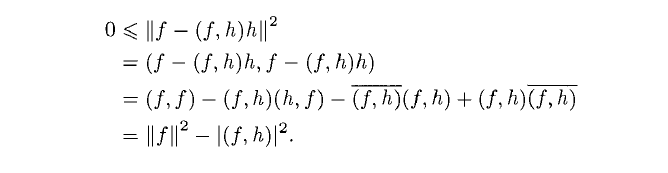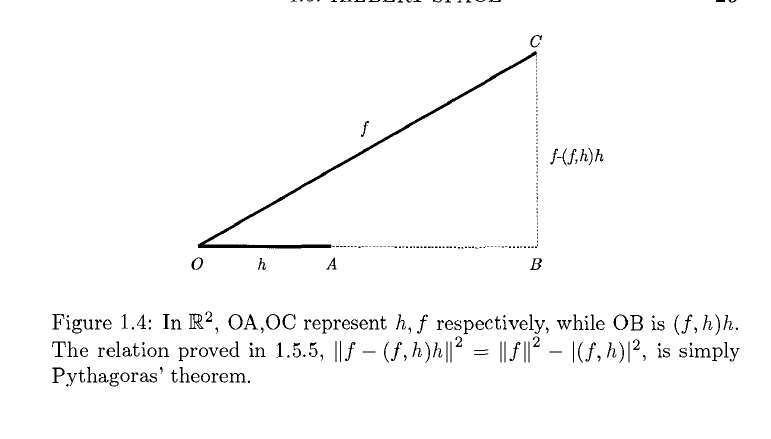# 2 Thursday, January 13

My notes:

• $$K \subseteq {\mathcal{H}}$$ is complete iff $$K^\perp = 0$$.
• Bessel: for $$f\in {\mathcal{H}}$$ write $$f_n \mathrel{\vcenter{:}}={\left\langle {f},~{e_n} \right\rangle} e_n$$, then $${\left\lVert {(f_n)} \right\rVert}_{\ell^2({\mathbb{C}})} \leq {\left\lVert {f} \right\rVert}_{{\mathcal{H}}}^2$$.
• Best estimate: for any other sequence $$(c_n) \in \ell^2({\mathbb{C}}), {\left\lVert {f - \sum c_n e_n} \right\rVert} \geq {\left\lVert {f - \sum f_n e_n} \right\rVert}$$.
• For $$\left\{{e_n}\right\}$$ orthonormal, $$(c_n) \in \ell^2({\mathbb{C}}) \iff \sum c_n e_n$$ converges. If the series converges, it can be rearranged.
• Differentiating through an integral: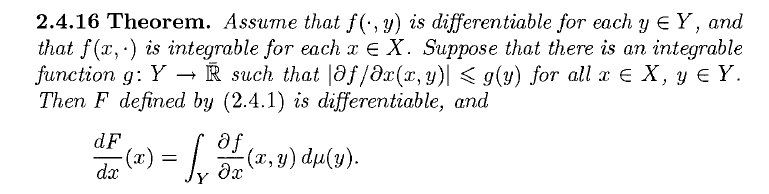• Parseval, Plancherel, and Fourier inversion: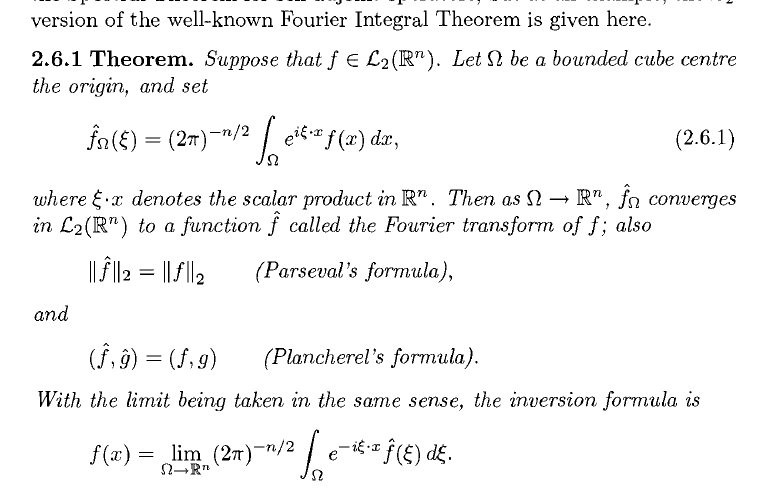Last time: any norm yields a metric: $$d(f, g) \mathrel{\vcenter{:}}={\left\lVert {f-g} \right\rVert}$$.

• Open/closed balls: $$B_r(f) \mathrel{\vcenter{:}}=\left\{{x{~\mathrel{\Big\vert}~}{\left\lVert {f-x} \right\rVert} < r}\right\}$$.
• Bounded subsets: contained in some ball of finite radius.
• $${\operatorname{diam}}S = \inf_{r, f} {\operatorname{diam}}B_r(f)$$ is the diameter of the smallest ball containing $$S$$.
• $$d(f, S) \mathrel{\vcenter{:}}=\inf_{x\in S} {\left\lVert {f-x} \right\rVert}$$.
• $$V = {\mathbb{R}}^n$$ with $${\left\lVert {f} \right\rVert}_2^2 \mathrel{\vcenter{:}}=\sum_{k\leq n} f_i^2$$, $$\mkern 1.5mu\overline{\mkern-1.5muB\mkern-1.5mu}\mkern 1.5mu_0(1)$$ is a metric space but not a vector space.
• For $$L^2$$, there are unique least squares projections, but uniqueness may fail for $$L^1$$.
• Counterexample: take a line $$M = \left\{{{\left[ {\alpha, \alpha} \right]}}\right\}$$ in $${\mathbb{R}}^2$$ of angle $$\pi/4$$ with respect to the $$x{\hbox{-}}$$axis and consider $$f\mathrel{\vcenter{:}}={\left[ {[} \right]}0, 1]$$. Then for $$g\mathrel{\vcenter{:}}=(\alpha, \alpha)$$, $${\left\lVert {f-g} \right\rVert} = {\left\lvert {1-\alpha} \right\rvert} + {\left\lvert {\alpha} \right\rvert} \geq {\left\lvert {1-\alpha + \alpha} \right\rvert} = 1$$, and the minimizer occurs for any $$\alpha \in [0, 1]$$.
• Similar issues may happen for $$L^\infty$$ – but $$L^1, L^\infty$$ have sharper tails than $$L^2$$, so this can be useful e.g. in image problems.
• If limits of sequences $$(f_n)$$ exist, i.e. $${\left\lVert {f_n - f_m} \right\rVert}\to 0$$, then the limiting function $$f_n\to f$$ is unique by the triangle inequality.
• Example from last time: $$\operatorname{diag}\qty{ 1, {1\over 2}, \cdots, {1\over n}} \to A$$ a compact self-adjoint operator with $$\operatorname{Spec}A = \left\{{1\over n}\right\}_{n\geq 0}$$.
• What is $$\ker A$$? Note that $$0\in \sigma(A)$$, where $$\sigma(A)$$ is the set where $$(A-I\lambda)^{-1}$$ is not defined. It turns out $$\ker A = \left\{{0}\right\}$$.
• Defining closures of subsets: for $$S \subseteq V$$, say $$f\in \mkern 1.5mu\overline{\mkern-1.5muS\mkern-1.5mu}\mkern 1.5mu$$ iff there exists a sequence of not necessarily distinct points $$f_n \in S$$ with $$f_n\to f$$.
• Say $$S_1 \subseteq S_2 \subseteq V$$ is closed in $$S_2$$ iff $$S_1 = C \cap S_2$$ for some $$C$$ closed in $$V$$. The closure of $$S_1$$ in $$S_2$$ is $${ \operatorname{cl}} _V(S_1) \cap S_2$$.
• A set that is neither open nor closed: $$X \mathrel{\vcenter{:}}=[a, b] \cap{\mathbb{Q}}$$, and $${{\partial}}X = [a,b] \supseteq X$$ is actually larger.
• Recall the little $$\ell^p$$ norms: $${\left\lVert {(f_n)} \right\rVert}_{p} \mathrel{\vcenter{:}}=\qty{ \sum {\left\lvert {f_n} \right\rvert}^p }^{1\over p}$$ and $${\left\lVert {(f_n)} \right\rVert}_{\infty} \mathrel{\vcenter{:}}=\sup_n {\left\lvert {f_n} \right\rvert}$$.
• Prove Jensen’s inequality for concave functions.
• Prove Young’s inequality.
• Prove Holder’s inequality.
• Idea: consider $$a = \widehat{f_n} \mathrel{\vcenter{:}}={\left\lvert {f_n} \right\rvert}/{\left\lVert {f_n} \right\rVert}^p, b = \widehat{g_n} \mathrel{\vcenter{:}}={\left\lvert {g_n} \right\rvert}/{\left\lVert {g_n} \right\rVert}_q$$ and apply Young’s after summing over $$n$$.
• Prove Minkowski’s inequality.
• Idea: use that $$(p-1)q=p$$ and apply the triangle inequality and then Holder to $$\sum {\left\lvert {f_n + g_n} \right\rvert}^p$$. Also use that $$q^{-1}= 1-p^{-1}$$, and divide through this inequality at the end. Be sure to check the cases $${\left\lVert {f+g} \right\rVert}_p = 0, \infty$$.

# 3 Tuesday, January 18

Last time:

• $${\left\lVert {f} \right\rVert}_{\ell^p} = \qty{\sum {\left\lvert {f_n} \right\rvert} ^p}^{1\over p}$$
• $${\left\lVert {f} \right\rVert}_{\ell^ \infty} = \sup_{n} {\left\lvert {f_n} \right\rvert}$$.

Today:

• $$\ell_p = \left\{{f \mathrel{\vcenter{:}}=(f_n) {~\mathrel{\Big\vert}~}{\left\lVert {f} \right\rVert}_{\ell^p} < \infty }\right\}$$.

• Example: set $$f^k \mathrel{\vcenter{:}}=(0, 0, \cdots, 1, 1, \cdots)$$ with zeros for the first $$k-1$$ entires and ones for all remaining entries. Then $$f^k_i \overset{k\to\infty}\longrightarrow 0$$ for each fixed component at index $$i$$. So $$f^k \to (0)$$ component-wise, but $${\left\lVert {f_k} \right\rVert}_{\ell^\infty} = 1$$ for every $$k$$, so this doesn’t converge in $$\ell_\infty$$.

• Recall the $${\varepsilon}{\hbox{-}}\delta$$ and limit definitions of continuity.

• Recall the definition of uniform continuity.

• For $$\Omega \subseteq {\mathbb{R}}^n$$, write $$C(\Omega)$$ for the $${\mathbb{R}}{\hbox{-}}$$vector space of continuous bounded functionals $$f: \Omega\to {\mathbb{C}}$$ with the norm $${\left\lVert {f} \right\rVert}_{L^\infty} = \sup_{x\in \Omega} {\left\lvert {f(x)} \right\rvert}$$.

• Define $$C^k(\Omega, {\mathbb{C}}^m)$$ to be functions with $$k$$ continuous partial derivatives which are bounded, and set $$C^\infty(\Omega, {\mathbb{C}}^m) = \cap_{k\geq 0} C^k(\Omega, {\mathbb{C}}^m)$$. Define a norm $${\left\lVert {f} \right\rVert}_{C^k} = \sum_{j\leq k} \sup_{x\in \Omega} {\left\lvert {f^{(j)} (x)} \right\rvert}$$.

• Take $$g(x) = 2-x^2$$ and consider $${\mathbb{B}}_{1\over 2}(g)$$ in $$C[0, 1]$$ with $${\left\lVert {{-}} \right\rVert}_{L^\infty}$$.

• Show that convergent implies Cauchy-convergent using the triangle inequality.

• Lipschitz with $${\left\lvert {c} \right\rvert} < 1$$ implies Cauchy.

• Lemma 1.4.2: $${\left\lVert {f_{n+k} - f_n} \right\rVert} \leq q^n (1-q)^{-1} {\left\lVert {f_1 - f_0} \right\rVert}$$ for all $$k\geq 0$$. Use \begin{align*} {\left\lVert {f_{n+k} - f_n} \right\rVert} = {\left\lVert {\sum_{j=1}^k (f_{n+j} - f_{n+j-1} ) } \right\rVert} \leq {\left\lVert {f_1 - f_0} \right\rVert} \sum_{j=1}^k q^{n+j-1} \overset{n\to\infty}\longrightarrow 0 .\end{align*}

• Counterexample, not all normed spaces are complete: take $$V = C[-1, 1]$$ with $${\left\lVert {f} \right\rVert}_{L^1} \mathrel{\vcenter{:}}=\int_{-1}^1 {\left\lvert {f(x) } \right\rvert}\,dx$$. Define a sequence of functions $$(f_n)$$: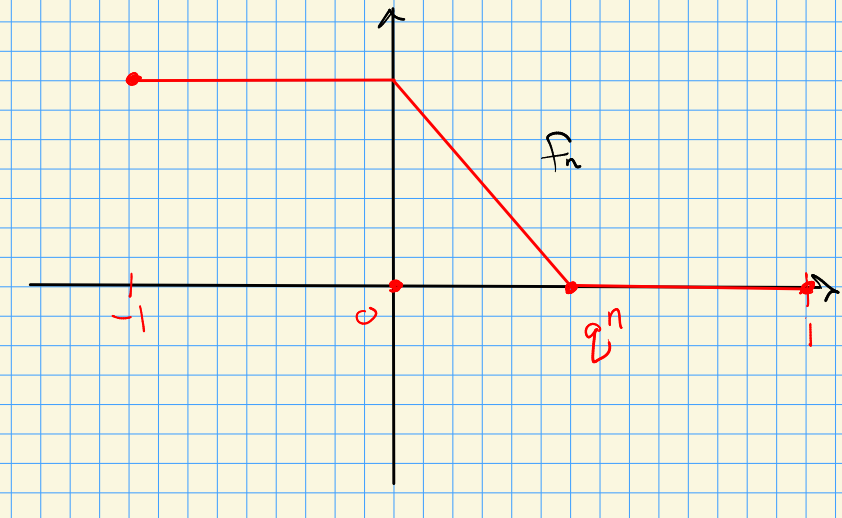Check that $${\left\lVert {f_{n+1} - f_n} \right\rVert}_{L^1} \leq q {\left\lVert {f_n - f_{n-1}} \right\rVert}_{L^1}$$, and pointwise $$f_n \to \chi_{[-1, 0]}$$ which is discontinuous and not in $$C[-1, 1]$$.

• Banach spaces: complete normed vector spaces.

• Series:

• Convergent: $$f \mathrel{\vcenter{:}}=\lim_N \sum_{n\leq N} f_n \in V$$.
• Absolute convergence: $$\sum {\left\lVert {f_n} \right\rVert} < \infty$$.
• In a Banach space, absolutely convergent series can be rearranged.
• Theorem: A normed space is complete iff absolute convergence $$\implies$$ convergence. Proof:

• Step 1: show that every Cauchy sequence has a convergent subsequence.
• Set $$a_n \mathrel{\vcenter{:}}=\sup_{m > n} {\left\lVert {f_n - f_m} \right\rVert}$$, then Cauchy implies $$a_n\to 0$$ in $${\mathbb{R}}$$.
• Get a convergent subsequence $$a_{n_j} \leq j^{-2}$$.
• Set $$g_j \mathrel{\vcenter{:}}= f_{n_j} - f_{n_{j+1}}$$, then $$g\mathrel{\vcenter{:}}=\sum g_j$$ absolutely converges, say to $$g$$.
• Note $$f_{n_i} - f_{n_{i+1}} = \sum_{j=1}^i g_j$$, so the subsequence $$(f_{n_j})$$ is convergent.
• Step 2: use this to show that the original sequence $$(f_n)$$ converges.
• Set $$f = \lim f_n$$, then $${\left\lVert {f_n - f} \right\rVert} \leq {\left\lVert {f_n - f_{n_i}} \right\rVert} + {\left\lVert {f_{n_i} - f} \right\rVert}$$, using Cauchy for the first $${\varepsilon}$$ and the convergent subsequence for the second.

Some theorems that hold in Hilbert spaces but not necessarily Banach spaces: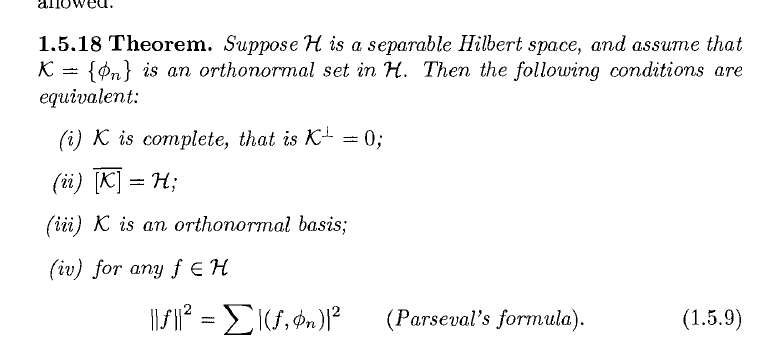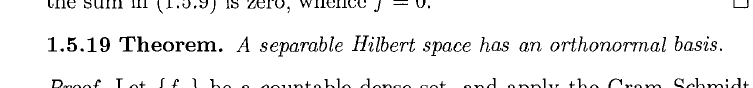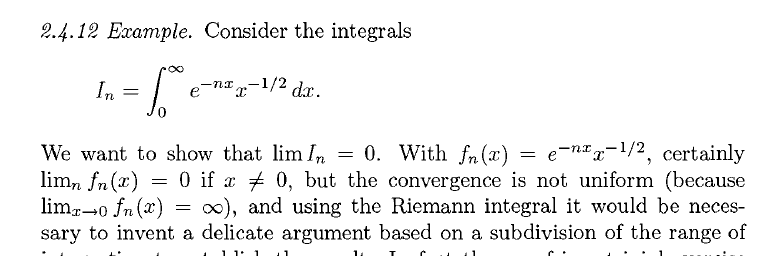Absolute continuity: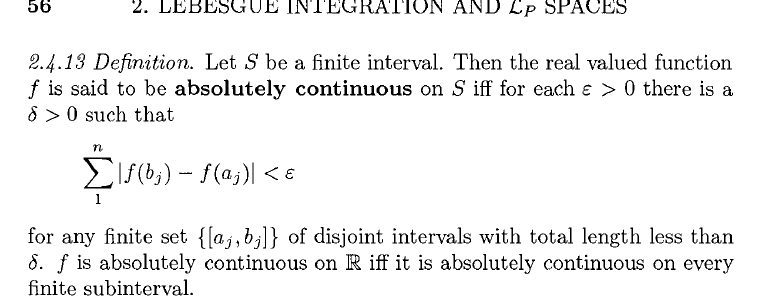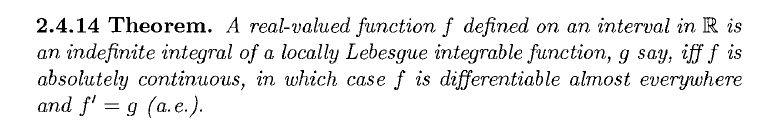$$L_p^{\mathsf{loc}}$$: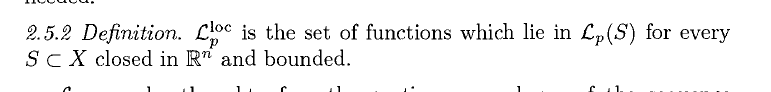$$\ker L = 0$$ may not be sufficient to guarantee bijectivity in infinite dimensions: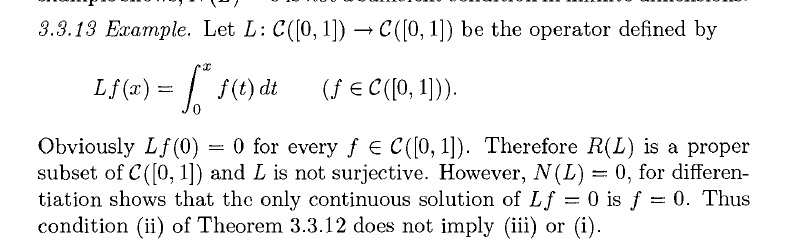Boundedness: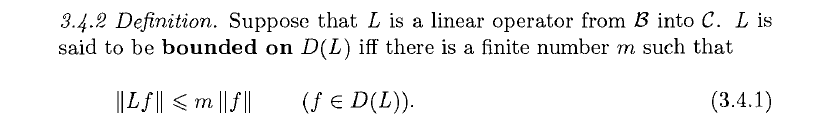Bounded iff continuous: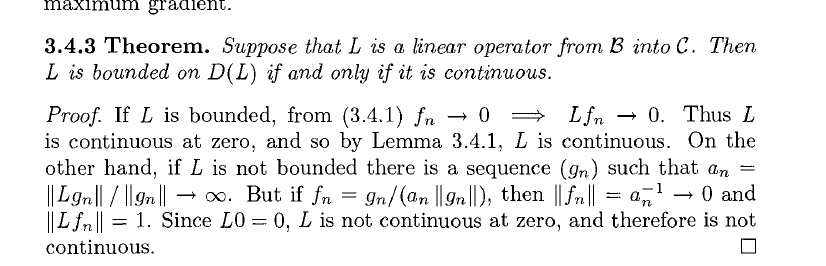# 4 More Banach Spaces (Thursday, January 20)

Last time: complete iff absolutely convergent implies convergent. Today: wrapping up some results on Banach and Hilbert spaces, skipping a review of $$L^p$$ spaces, and starting on operators next week.

Note that $$S \mathrel{\vcenter{:}}=(0, 1]$$ is open and not complete, but $${ \operatorname{cl}} _{\mathbb{R}}(S) = [0, 1]$$ is both closed and complete. Generalizing:

A subset $$S \subseteq B$$ of a Banach space is complete iff $$S$$ is closed in $$B$$.

$$\impliedby$$: If $$S$$ is closed, a Cauchy sequence $$(f_n)$$ in $$S$$ converges to some $$f\in B$$. Since $$S$$ is closed in $$B$$, in fact $$f\in S$$.

$$\implies$$: Suppose that for $$f\in { \operatorname{cl}} _B(S)$$, there is a Cauchy sequence $$f_n\to f$$ with $$f_n \in S$$ and $$f\in B$$. Since $$S$$ is complete, $$f\in S$$, so $${ \operatorname{cl}} _B(S) \subseteq S$$ making $$S$$ closed.

For $$\Omega \subseteq {\mathbb{R}}^n$$, the space $$X = (C(\Omega; {\mathbb{C}}), {\left\lVert {{-}} \right\rVert}_\infty)$$ is a Banach space.

This is not complete with respect to any other $$L^p$$ norm with $$p<\infty$$!

Use the lemma – write $$B$$ for the space of all bounded (not necessarily continuous) functions on $$\Omega$$, which is clearly a normed vector space, so it suffice to show

• $$X \subseteq B$$ is closed,
• $$B$$ is a Banach space (i.e. complete).

Step 1: we’ll show $${ \operatorname{cl}} _B(X) \subseteq X$$. Take $$f$$ to be a limit point of $$X$$, then for every $${\varepsilon}>0$$ there is a $$g\in X$$ with $${\left\lVert {f-g} \right\rVert} < {\varepsilon}$$. Apply the triangle inequality: \begin{align*} {\left\lVert {f} \right\rVert} \leq {\left\lVert {f-g+g} \right\rVert}\leq {\left\lVert {f-g} \right\rVert} + {\left\lVert {g} \right\rVert} = {\varepsilon}+ C < \infty ,\end{align*} so $$f\in { \operatorname{cl}} _B(X) \subseteq B$$ since it is bounded. It remains to show $$f$$ is continuous. Use that $${\left\lVert {f-g} \right\rVert}\infty <{\varepsilon}$$ and continuity of $$g$$ to get $${\left\lvert {g(x) - g(x_0)} \right\rvert} < {\varepsilon}$$ for $${\left\lvert {x-x_0} \right\rvert}<{\varepsilon}$$. Now \begin{align*} {\left\lvert {f(x) - f(x_0)} \right\rvert} &= {\left\lvert {f(x) - g(x) + g(x) -g(x_0) + g(x_0) - f(x_0)} \right\rvert} \\ &\leq {\left\lvert {f(x) - g(x)} \right\rvert} + {\left\lvert {g(x) - g(x_0)} \right\rvert} + {\left\lvert {f(x_0) - g(x_0)} \right\rvert} \\ &\leq 3{\varepsilon} .\end{align*} So $$X$$ is closed in $$B$$.

Step 2: Let $$f_n$$ be Cauchy in $$B$$, and note that we have a pointwise bound $${\left\lvert {f_n(x) - f_n(x_0)} \right\rvert} \leq {\left\lVert {f_n - f_m} \right\rVert} \to 0$$. So pointwise, $$f_n(x)$$ is a Cauchy sequence in $${\mathbb{C}}$$ which is complete, so $$f_n(x) \to f(x)$$ for some $$f: \Omega\to {\mathbb{C}}$$. We now want to show $$f_n\to f$$ in $$X$$. Using that $$f_n$$ is Cauchy in $$X$$, produce an $$N_0$$ such that $$n, m\geq N_0 \implies {\left\lVert {f_n - f_m} \right\rVert}< {\varepsilon}$$. Now \begin{align*} {\left\lVert {f - f_n} \right\rVert} &= \sup_{x\in \Omega} {\left\lvert {f(x) - f_n(x)} \right\rvert} \\ &\leq \sup_{x\in \Omega} \sup_{m\geq N_0} {\left\lvert {f_m(x) - f_n(x)} \right\rvert} \\ &= \sup_{m\geq N_0} \sup_{x\in \Omega} {\left\lvert {f_m(x) - f_n(x)} \right\rvert} \\ &= \sup_{m\geq N_0} {\left\lVert {f_m - f_n} \right\rVert} \\ &\leq {\varepsilon} .\end{align*} Now use the reverse triangle inequality to show $$f_n$$ is bounded \begin{align*} {\left\lVert {f} \right\rVert} - {\left\lVert {f_n} \right\rVert} \leq {\left\lVert {f-f_n} \right\rVert} < {\varepsilon}\implies {\left\lVert {f} \right\rVert} < \infty .\end{align*}

Now by problem 1.13, every Cauchy sequence is bounded, so $$f_n \to f\in B$$.

Extending to vector-valued functions: for $$\Omega \subseteq {\mathbb{R}}^n$$, take $$\mathbf{x} = {\left[ {x_1, \cdots, x_n} \right]}$$ and $$F = {\left[ {f_1, \cdots, f_m} \right]}: {\mathbb{C}}^n\to {\mathbb{C}}^m$$. Then there is a Banach space \begin{align*} X = C^(\Omega, {\mathbb{C}}^m), \qquad {\left\lVert {f} \right\rVert}_{C_1(\Omega)} \mathrel{\vcenter{:}}=\sum_{i\leq m} \sup_{x\in \Omega} {\left\lvert {f(x)} \right\rvert} + \sum_{i\leq m, j\leq n} \sup_{x\in \Omega} {\left\lvert {{\frac{\partial f_i}{\partial x_j}\,}(x) } \right\rvert} .\end{align*} Similarly define $$L^p(\Omega)$$, noting that $${\left\lVert {f} \right\rVert}_{L^\infty(\Omega)}$$ is the essential supremum.

For $$p\in (1, \infty)$$, the sequence space $$X = (\ell^p, {\left\lVert {{-}} \right\rVert}_{\ell^p})$$ is a Banach space.

A closed subspace of a Banach space is a linear subspace $$M \leq B$$ which is norm-closed in $$B$$.

\begin{align*} M \mathrel{\vcenter{:}}=\ker { \nabla\cdot }= \left\{{f\in C(\Omega) {~\mathrel{\Big\vert}~}{ \nabla\cdot }f = 0}\right\} \leq C(\Omega) \end{align*} is closed, where $${ \nabla\cdot }f$$ is the divergence of a function $$f$$.

For any $$S \subseteq B$$, one can also take the corresponding closed subspace $$\mkern 1.5mu\overline{\mkern-1.5mu[S]\mkern-1.5mu}\mkern 1.5mu \mathrel{\vcenter{:}}={ \operatorname{cl}} _B \mathop{\mathrm{span}}_{\mathbb{C}}\left\{{s\in S}\right\}$$, i.e. all linear combinations of elements in $$S$$ and their limits. This is called the closed linear span of $$S$$.

Let $$B = ( C[a, b], {\left\lVert {{-}} \right\rVert}_{L^\infty}$$ and for $$x_0 \in [a, b]$$ define \begin{align*} M \mathrel{\vcenter{:}}=\left\{{f\in C[a, b] {~\mathrel{\Big\vert}~}f(x_0) = 0}\right\}, \qquad N \mathrel{\vcenter{:}}=\left\{{f\in C[a, b] {~\mathrel{\Big\vert}~}f(x_0) \leq c}\right\} .\end{align*} Show that these are closed subspaces with no nontrivial open subsets of $$B$$, since any $$f\in M$$ can be perturbed to be nonzero at $$x_0$$ with an arbitrarily small norm difference.

Recall that for $$S_1 \subseteq S_2 \subseteq B$$, $$S_1$$ is dense in $$S_2$$ iff $${ \operatorname{cl}} _{S_2}(S_1) = S_2$$. Recall Weierstrass’ theorem: for $$\Omega \subseteq {\mathbb{R}}^n$$ is closed and bounded and write $${\mathcal{O}}\mathrel{\vcenter{:}}={\mathbb{R}}[x_1, \cdots, x_n]$$ for the polynomials in $$n$$ variables. Then $${\mathcal{O}}\subseteq C(\Omega)$$, and $${ \operatorname{cl}} _{C(\Omega)} {\mathcal{O}}= C(\Omega)$$, i.e. $${\mathcal{O}}$$ is a dense subspace in the $$L^\infty$$ norms. In fact, piecewise linear functions are dense.

Norms are equivalent iff $$c_1 {\left\lVert {f} \right\rVert}_a \leq {\left\lVert {f} \right\rVert}_b\leq c_2 {\left\lVert {f} \right\rVert}_a$$ for some constants $$c_i$$. All norms on $${\mathbb{R}}^n$$ (resp. $${\mathbb{C}}^n$$) are equivalent.

For $$a>0, f\in C[0, 1]$$, define \begin{align*} {\left\lVert {f} \right\rVert} \mathrel{\vcenter{:}}=\sup_{x\in I} {\left\lvert {e^{-ax} f(x)} \right\rvert} ,\end{align*} which can be thought of as a weighting on the uniform norm which de-emphasizes the tails of functions near the endpoints. This is equivalent to $${\left\lVert {{-}} \right\rVert}_\infty$$, since \begin{align*} e^a \sup {\left\lvert {f} \right\rvert} \leq \sup {\left\lvert {e^{-ax} f} \right\rvert} \leq 1\cdot \sup {\left\lvert {f} \right\rvert} .\end{align*}

Note that a basis for a norm can be used as a basis with respect to an equivalent norm in finite dimensions. In infinite dimensions this may not hold – e.g. for Fourier series, $$\left\{{e_k(x)}\right\}_{k\in {\mathbb{Z}}}$$ is not a basis for $$C[0, 2\pi]$$ with the sup norm.

An $$B\in \mathcal{B}$$ is separable iff $$X$$ contains a countable dense subset $$S = \left\{{f_k}\right\}_{k\geq 0}$$ such that for each $$f\in B$$ and $${\varepsilon}>0$$, there is an $$f_k\in S$$ with $${\left\lVert {f-f_k} \right\rVert} < {\varepsilon}$$.

Show that

• $$\Omega \subseteq {\mathbb{R}}^n$$ a bounded subset, $$C(\Omega), {\left\lVert {{-}} \right\rVert}_{\sup}$$ is separable.
• $$\ell^p$$ is separable for $$p\in (1,\infty)$$.
• $$\ell^\infty$$ is not separable.

## 4.1 Random Notes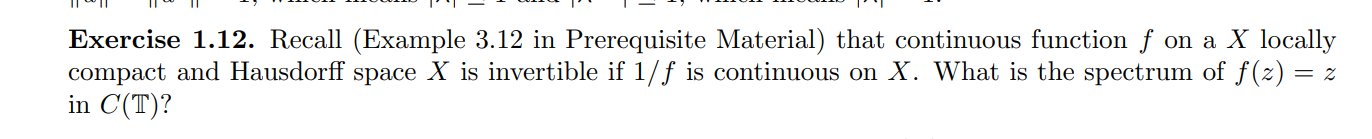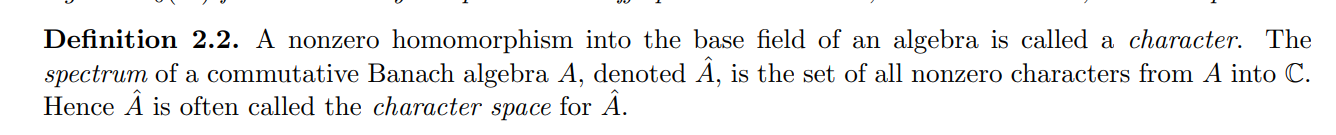# 5 Tuesday, January 25

• Inner products:
• $${\left\langle {f},~{f} \right\rangle} \geq 0$$ and $${\left\langle {f},~{f} \right\rangle} = 0 \iff f=0$$
• $${\left\langle {f},~{g+h} \right\rangle} = {\left\langle {f},~{g} \right\rangle} + {\left\langle {f},~{h} \right\rangle}$$
• $${\left\langle {f},~{g} \right\rangle} = \mkern 1.5mu\overline{\mkern-1.5mu{\left\langle {g},~{f} \right\rangle}\mkern-1.5mu}\mkern 1.5mu$$
• $${\left\langle {\alpha f},~{g} \right\rangle} = \alpha {\left\langle {f},~{g} \right\rangle}$$
• A pre-Hilbert space is an inner-product space.
• Example: $${\left\langle {f},~{g} \right\rangle} \mathrel{\vcenter{:}}=\int_\Omega w(x) f(x) \mkern 1.5mu\overline{\mkern-1.5mug(x)\mkern-1.5mu}\mkern 1.5mu\,dx$$ for $$f,g\in C(\Omega)$$, where $$w$$ is any weighting function.
• Example: $${\left\langle {f},~{g} \right\rangle} = \sum f_i \mkern 1.5mu\overline{\mkern-1.5mug_i\mkern-1.5mu}\mkern 1.5mu$$ for $$f,g\in {\mathbb{C}}^n$$.
• Inner products induce norms: $${\left\lVert {f} \right\rVert} \mathrel{\vcenter{:}}=\sqrt{{\left\langle {f},~{f} \right\rangle}}$$
• Orthogonality: write $$f\perp g$$ iff $${\left\langle {f},~{g} \right\rangle} = 0$$ and $$S^\perp = \left\{{f\in {\mathcal{H}}{~\mathrel{\Big\vert}~}f\perp s \, \forall s\in S}\right\}$$.
• Definition of a Hilbert space: a pre-Hilbert space complete with respect to the norm induced by its inner product.
• Recall $$C(\Omega)$$ with $${\left\langle {f},~{g} \right\rangle} \mathrel{\vcenter{:}}=\int_\Omega f\mkern 1.5mu\overline{\mkern-1.5mug\mkern-1.5mu}\mkern 1.5mu$$ is not complete, thus not a Hilbert space.
• Example: a common optimization problem, $$\mathrm{argmin} {\left\lVert {x} \right\rVert}$$ such that $$Ax=0$$.

\begin{align*} {\left\lvert {{\left\langle {f},~{g} \right\rangle}} \right\rvert}\leq {\left\lVert {f} \right\rVert} {\left\lVert {g} \right\rVert} .\end{align*}

Use $${\left\langle {f},~{g} \right\rangle} = {\left\lvert {f} \right\rvert} {\left\lvert {g} \right\rvert} \cos \theta_{fg}$$ where $${\left\lvert { \cos \theta } \right\rvert} \leq 1$$. - Assume $$g\neq 0$$, then STS $${\left\langle {f},~{g\over {\left\lVert {g} \right\rVert}} \right\rangle} \leq {\left\lVert {f} \right\rVert}$$. - Use \begin{align*} 0 &\leq {\left\lVert {f - {\left\langle {f},~{g} \right\rangle} g} \right\rVert}^2 \\ &= {\left\langle {f - {\left\langle {f},~{g} \right\rangle} g},~{f - {\left\langle {f},~{g} \right\rangle} g} \right\rangle} \\ &= {\left\langle {f},~{f} \right\rangle} - {\left\langle {f},~{g} \right\rangle} {\left\langle {g},~{f} \right\rangle} - \mkern 1.5mu\overline{\mkern-1.5mu{\left\langle {f},~{g} \right\rangle}\mkern-1.5mu}\mkern 1.5mu {\left\langle {f},~{g} \right\rangle} + {\left\langle {f},~{g} \right\rangle} \mkern 1.5mu\overline{\mkern-1.5mu{\left\langle {f},~{g} \right\rangle} \mkern-1.5mu}\mkern 1.5mu \\ &= {\left\lVert {f} \right\rVert}^2 - {\left\lvert { {\left\langle {f},~{g} \right\rangle}} \right\rvert}^2 .\end{align*}

Let $$f\in M\leq {\mathcal{H}}$$ be a closed (and thus complete) subspace of a Hilbert space $${\mathcal{H}}$$. Then there is a unique element $$g$$ in $${\mathcal{H}}$$ closest to $$M$$ in the norm.

Let $$d\mathrel{\vcenter{:}}=\operatorname{dist}(f, M)$$, choose a sequence $$g_n \in M$$ such that $${\left\lVert {f-g_n} \right\rVert}\to d$$, which is possible since $$d = \inf_{g\in M}{\left\lVert {f-g} \right\rVert}$$. Apply the parallelogram law to write \begin{align*} {\left\lVert {g_n - g_m} \right\rVert}^2 &= {\left\lVert {(g_n - f) - (g_m - f) } \right\rVert}^2 \\ &= 2{\left\lVert {g_n - f} \right\rVert}^2 + 2{\left\lVert {g_m - f} \right\rVert}^2 - 4{\left\lVert { {1\over 2} (g_n + g_m) - f} \right\rVert}^2 \\ &\leq 2{\left\lVert {g_n - f} \right\rVert}^2 + 2{\left\lVert {g_m - f} \right\rVert}^2 - 4d^2 \\ &\leq 2d^2 + 2d^2 - 4d^2 \\ &= 0 ,\end{align*} so the $$g_n$$ are Cauchy. Here we’ve used that $${1\over 2}(g_n + g_m) = m \in M$$ since $$M$$ is a subspace, and $${\left\lVert {m-f} \right\rVert} \geq d$$. Since $$M$$ is complete, $$g_n\to g\in M$$ and moreover $${\left\lVert {f-g} \right\rVert} = d$$. For uniqueness, if $${\left\lVert {f-g'} \right\rVert} = d$$ then \begin{align*} {\left\lVert { f - {1\over 2} (g+g')} \right\rVert}^2 = d^2 - {\left\lVert {g-g'} \right\rVert}^2 < d^2 \qquad \contradiction .\end{align*}

Let $$M\leq {\mathcal{H}}$$ be a closed subspace of a Hilbert space. Then $$M^\perp \leq {\mathcal{H}}$$ is closed, and $${\mathcal{H}}= M \oplus M^\perp$$. In the decomposition $$f= g+h$$, in fact $$g\in M$$ is the closest approximation to $$f$$ in $$M$$, making this decomposition unique.

STS $${\mathcal{H}}= M + M^\perp$$ by the exercises. If $$f\in M$$, take $$f=g+h$$ where $$g=f$$ and $$h=0$$, so suppose $$f\not\in M$$. Let $$g = \mathrm{argmin} \operatorname{dist}(f, M) \in M$$, and we claim $$f-g\in M^\perp$$, so $${\left\langle {f-g},~{h} \right\rangle} = 0$$ for any $$h\in M$$. For all $$h\in M$$ and $$\alpha > 0$$, we have $$g + \alpha h\in M$$, so \begin{align*} {\left\lVert {f - g} \right\rVert}^2 &\leq {\left\lVert {f - (g+\alpha h)} \right\rVert}^2 \\ &= {\left\lVert {f-g} \right\rVert}^2 -2\Re\alpha {\left\langle {h},~{f-g} \right\rangle} + \alpha^2 {\left\lVert {h} \right\rVert}^2 ,\end{align*} so \begin{align*} 2\Re \alpha {\left\langle {h},~{f-g} \right\rangle} \leq \alpha^2 {\left\lVert {h} \right\rVert}^2 \implies 2\Re {\left\langle {h},~{f-g} \right\rangle} \leq \alpha {\left\lVert {h} \right\rVert}^2 \overset{\alpha\to 0}\longrightarrow 0 ,\end{align*} so $$\Re{\left\langle {h},~{f-g} \right\rangle} = 0$$. Similarly $$\Im{\left\langle {h},~{f-g} \right\rangle} = 0$$.

Show $$S^\perp$$ is closed for any $$S\in {\mathcal{H}}$$, and in fact $$S^\perp = \mathop{\mathrm{span}}_{\mathbb{C}}({ \operatorname{cl}} _{\mathcal{H}}(S))^\perp$$, and if $$f\in S \cap S^\perp$$ then $$f=0$$.

Prove the parallelogram law \begin{align*} {\left\lVert {f-g} \right\rVert}^2 + {\left\lVert {f+g} \right\rVert}^2 = 2{\left\lVert {f} \right\rVert}^2 + 2{\left\lVert {g} \right\rVert}^2 .\end{align*}

# 6 Thursday, January 27

Notes:

• Bessel: \begin{align*} \sum_n {\left\lvert { {\left\langle {f},~{\phi_n} \right\rangle} } \right\rvert} \leq {\left\lVert {f} \right\rVert}^2,\qquad {\left\lVert {f} \right\rVert} \mathrel{\vcenter{:}}=\sqrt{{\left\langle {f},~{f} \right\rangle}} .\end{align*}
• Prove using the fact that \begin{align*} 0\leq {\left\lVert {f - \sum_{n\leq m} {\left\langle {f},~{\phi_n} \right\rangle} \phi_n } \right\rVert}^2 = {\left\lVert {f} \right\rVert}^2 - \sum_{n\leq m} {\left\lvert {{\left\langle {f},~{\phi_n} \right\rangle}} \right\rvert}^2 .\end{align*}
• Best fit: \begin{align*} {\left\lVert {f - \sum_{n\leq m} c_n \phi_n } \right\rVert} \geq {\left\lVert {f - \sum_{n\leq m} {\left\langle {f},~{\phi_n} \right\rangle} \phi_n } \right\rVert} ,\end{align*} so define projections $$P_M(f) \mathrel{\vcenter{:}}=\sum {\left\langle {f},~{\phi_n} \right\rangle} \phi_n$$ for $$\mathop{\mathrm{span}}\left\{{\phi_n}\right\} = M$$.
• Prove using \begin{align*} {\left\lVert {f - \sum_{n\leq m} c_n \phi_n } \right\rVert} &= {\left\lVert {f} \right\rVert}^2 - \sum_{n\leq m} {\left\lvert {{\left\langle {f},~{\phi_n} \right\rangle}} \right\rvert}^2 + \sum_{n\leq m} {\left\lvert {{\left\langle {f},~{\phi_n} \right\rangle} - c_n } \right\rvert}^2 \\ &\geq {\left\lVert {f} \right\rVert}^2 - \sum_{n\leq m} {\left\lvert {{\left\langle {f},~{\phi_n} \right\rangle}} \right\rvert}^2 .\end{align*}
• Hilbert spaces are separable: have countable dense subsets.
• $$\ell^\infty({\mathbb{Z}})$$ is not separable.
• For $$\left\{{\phi_n}\right\}$$ orthonormal and $$\left\{{c_n}\right\}$$ scalars, $$\sum c_n \phi_n$$ is convergent iff $$\left\{{a_n}\right\}\in \ell^2({\mathbb{Z}})$$, so $$\sum{\left\lvert {c_n} \right\rvert}^2<\infty$$. If it converges, it can be rearranged, and \begin{align*} {\left\lVert {\sum c_n \phi_n} \right\rVert}^2 = {\left\lVert {\left\{{c_n}\right\}} \right\rVert}_{\ell^2({\mathbb{Z}})}^2 = \sum {\left\lvert {c_n} \right\rvert}^2 .\end{align*}
• To prove, use that $${\left\lVert { \sum_{i\leq n\leq j}c_n \phi_n} \right\rVert}^2 = \sum_{i\leq n\leq j}{\left\lvert {c_n} \right\rvert}^2$$, so the sequence $$\left\{{S_m}\right\}_{m\geq 0}$$ where $$S_m \mathrel{\vcenter{:}}=\sum_{n\leq m} c_n\phi_n$$ is Cauchy since $$\sum{\left\lvert {c_n} \right\rvert}^2$$ converges.
• If $$g = \sum c_n \phi_n$$ and $$f = \sum c_{m_n} \phi_{m_n}$$ is a rearrangement, if $$\left\{{c_n}\right\}\in \ell^2$$ then $${\left\lVert {f} \right\rVert}^2 = {\left\lVert {g} \right\rVert}^2 = \sum {\left\lvert {c_n} \right\rvert}^2$$. Then $${\left\lVert {f-g} \right\rVert}^2 = {\left\lVert {f} \right\rVert}^2 + {\left\lVert {g} \right\rVert}^2 - 2\Re{\left\langle {f},~{g} \right\rangle}$$, but $${\left\langle {f},~{g} \right\rangle} = \sum {\left\lvert {c_n} \right\rvert}^2$$, so $${\left\lVert {f-g} \right\rVert} = 0$$.
• If $$K = \left\{{\phi_n}\right\} \subseteq {\mathcal{H}}$$ is a proper subset, so $$\mathop{\mathrm{span}}\left\{{\phi_n}\right\}\neq {\mathcal{H}}$$, write $$P_K(f) = \sum {\left\langle {f},~{\phi_n} \right\rangle}\phi_n$$. Then $$P_K(f) = 0$$ if $$f\in { \operatorname{cl}} (K)^\perp$$, $$P_K(f) = f$$ if $$f\in { \operatorname{cl}} (K)$$, and if $$f\not \in K$$, since $${ \operatorname{cl}} (K)\leq {\mathcal{H}}$$ is closed then there exists a $$g\in { \operatorname{cl}} (K)$$ where $${\left\lVert {f-g} \right\rVert} = \operatorname{dist}(f, K)$$. Write $$f = h + (f-g) \in { \operatorname{cl}} (K) \oplus { \operatorname{cl}} (K)^\perp$$, so $$f = P_K f + (I-P_K)f$$.
• Recall complete subspaces of Banach spaces are closed.
• Next theorem: every separable Hilbert space admits an orthonormal basis.

If $${\mathcal{H}}$$ is a separable Hilbert spaces and $$K = \left\{{\phi_n}\right\}$$ is an orthonormal set, then TFAE

• $$K$$ is complete, i.e. $$K^\perp = 0$$ (taking the closure is not needed),
• $${ \operatorname{cl}} {\mathop{\mathrm{span}}K} = {\mathcal{H}}$$,
• $$K$$ is an orthonormal basis,
• For all $$f\in {\mathcal{H}}$$, $${\left\lVert {f} \right\rVert} = \sum_{n\geq 0} {\left\lvert {{\left\langle {f},~{\phi_n} \right\rangle}} \right\rvert}^2$$ (Parseval).

Note $${\left\langle {f},~{\phi_n} \right\rangle}$$ is the $$n$$th Fourier coefficient $$\widehat{f}(\xi) = \sum {\left\langle {f},~{\phi_n} \right\rangle}\phi_n(\xi)$$, and Parseval says $${\left\lVert {f} \right\rVert}^2 = {\left\lVert {\widehat{f}} \right\rVert}^2$$.

$$1\implies 2$$: Let $$f\in {\mathcal{H}}\setminus{ \operatorname{cl}} \mathop{\mathrm{span}}(K)$$ and project, so $$f= g+h$$ with $$g,h\neq 0$$. But $$g\in { \operatorname{cl}} \mathop{\mathrm{span}}(K)$$ and $$h\in { \operatorname{cl}} \mathop{\mathrm{span}}(K)^\perp = 0$$, forcing $$K^\perp = { \operatorname{cl}} (K)^\perp \neq 0$$. $$\contradiction$$

$$2\implies 3$$: Follows directly from previous lemma that $$f = P_Kf + (I-P_K)f$$.

$$3\implies 4$$: Write $$f\in {\mathcal{H}}$$ as $$f = \sum {\left\langle {f},~{\phi_n} \right\rangle} \phi_n$$ by sending $$m\to \infty$$ in the previous lemma.

$$4\implies 1$$: Toward a contradiction, suppose $$f\neq 0\in K^\perp$$. Then $${\left\lVert {f} \right\rVert} \neq 0$$ but $${\left\langle {f},~{\phi_n} \right\rangle}=0$$ for all $$n$$, contradicting Parseval. $$\contradiction$$

# 7 Tuesday, February 01

Notes:

• Assume $${\mathcal{H}}$$ is a separable Hilbert space: there exists a countable set of vectors $$\left\{{v_i}\right\}_{i\in {\mathbb{Z}}}$$ which span a subspace that is dense in $${\mathcal{H}}$$, so $${ \operatorname{cl}} (\mathop{\mathrm{span}}\left\{{v_i}\right\}) = {\mathcal{H}}$$.
• Complete subspaces: $$M\leq {\mathcal{H}}$$ with $$M^\perp = 0$$.
• Show that for any $$S \subseteq {\mathcal{H}}$$, $$S^\perp$$ is closed in $${\mathcal{H}}$$, $$S^\perp = \qty{{ \operatorname{cl}} \mathop{\mathrm{span}}S}^\perp$$, and $$f\in S \cap S^\perp \implies f=0$$.
• If $$K = \left\{{\phi_k}\right\}_{k\in {\mathbb{Z}}}$$ is an orthonormal set in $${\mathcal{H}}$$, then TFAE
• $$K \leq {\mathcal{H}}$$ is a complete subspace, so $$K^\perp = 0$$, i.e. $${\left\langle {f},~{\phi_k} \right\rangle} = 0$$ for all $$k$$ implies $$f=0$$.
• $${ \operatorname{cl}} \mathop{\mathrm{span}}K = {\mathcal{H}}$$, so every $$f\in {\mathcal{H}}$$ is the limit of a sequence of vectors from $$\mathop{\mathrm{span}}K$$.
• $$K$$ is an orthonormal basis
• Parseval: equality in Bessel, i.e. $${\left\lVert {f} \right\rVert} = \sum {\left\lvert { {\left\langle {f},~{\phi_k} \right\rangle} } \right\rvert}^2$$
• Lemma: if $$M, N\leq {\mathcal{H}}$$ with $$\dim M < \dim N$$, then $$M^\perp \cap N \neq 0$$.
• Without loss of generality assume $$\dim N = n + 1$$ where $$n\mathrel{\vcenter{:}}=\dim M$$, take a basis $$\left\{{\psi_k}\right\}_{k\leq n+1}$$ for $$N$$.
• Try to find $$f\in N$$ with $$f\perp M$$, i.e. coefficients $$\left\{{b_i}\right\}_{i\leq n}$$ with $$\sum b_i \psi_i \perp \phi_k$$ for every $$\phi_k$$ basis elements of $$M$$.
• This is a linear system of $$n$$ equations in $$n+1$$ unknowns, so it has a nontrivial solution.
• Theorem, orthonormal bases are stable: if $$\left\{{\phi_k}\right\}$$ is an orthonormal basis and $$\left\{{\psi_k}\right\}$$ is an orthonormal system, if $$\sum {\left\lVert {\phi_k - \psi_k} \right\rVert}^2 < \infty$$ then $$\left\{{\psi_k}\right\}$$ is a basis.
• Assume note, then find a $$\psi_0 \in {\mathcal{H}}$$ with $${\left\lVert {\psi_0} \right\rVert} = 1$$ and $${\left\langle {\psi_0},~{\psi_j} \right\rangle} = 0$$ for all $$j$$.
• Choose $$N\gg 1$$ so that $$\sum_{k\geq N} {\left\lVert {\psi_k - \phi_k} \right\rVert} < 1$$.
• Use previous lemma to produce $$w\in \mathop{\mathrm{span}}\left\{{\psi_0, \psi_1,\cdots, \psi_N}\right\}$$ with $$w\neq 0$$ and $$w\perp \phi_j$$ for all $$j\leq N$$.
• Note $$w\perp \mathop{\mathrm{span}}\left\{{\psi_n}\right\}_{n > N}$$.
• Apply Parseval: \begin{align*} 0 &< {\left\lVert {w} \right\rVert}^2 \\ &= \sum_{n\geq 1} {\left\lvert {{\left\langle {w},~{\phi_n} \right\rangle}} \right\rvert}^2 \\ &= \sum_{n\geq N+1}{\left\lvert {{\left\langle {w},~{\phi_n} \right\rangle}} \right\rvert}^2 \\ &= \sum_{n\geq N+1} {\left\lvert {{\left\langle {w},~{\phi_n - \psi_n} \right\rangle}} \right\rvert}^2 \\ &\leq {\left\lVert {w} \right\rVert}^2 \sum_{n\geq N+1}{\left\lVert {\phi_n - \psi_n} \right\rVert}^2 \\ &< {\left\lVert {w} \right\rVert}^2 \cdot 1 ,\end{align*} where we’ve used that $${\left\langle {w},~{\psi_n} \right\rangle} = 0$$ for $$n\geq N$$. $$\contradiction$$
• $${\mathcal{H}}$$ admits a countable orthonormal basis iff $${\mathcal{H}}$$ is separable.
• $$\implies$$: clear, since the basis is countable, and every element is a limit of partial sums against the basis.
• $$\impliedby$$: Gram-Schmidt.
• $$h_1 = \psi_1$$ and $$\phi_1 = h_1/{\left\lVert {h_1} \right\rVert}$$
• $$h_n = \psi_n - \sum_{1\leq k\leq n-1} {\left\langle {\psi_k},~{\phi_k} \right\rangle} \phi_k$$ and normalize.
• Exercise: a closed subspace of a separable Hilbert space is separable.
• Linearly isometric inner product spaces: $$E\sim F$$ iff there is a map $$A: E\twoheadrightarrow F$$ with
• $$A(au + bv) = aAu + bAv$$
• $${\left\lVert {Au} \right\rVert}_F = {\left\lVert {u} \right\rVert}_E$$
• Theorem: if $${\mathcal{H}}_1, {\mathcal{H}}_2$$ are infinite dimensional separable Hilbert spaces, then $${\mathcal{H}}_1 \sim {\mathcal{H}}_2$$. Thus for any Hilbert space $${\mathcal{H}}$$ over $${\mathbb{C}}$$, $${\mathcal{H}}\sim \ell^2({\mathbb{C}})$$.
• Pick orthonormal bases $$\left\{{\phi_k}\right\} \subseteq {\mathcal{H}}_1, \left\{{\psi_k}\right\} \subseteq {\mathcal{H}}_2$$.
• For $$u\in {\mathcal{H}}_1$$, define $$Au \mathrel{\vcenter{:}}=\sum {\left\langle {u},~{\phi_k} \right\rangle}\psi_k$$, which converges – this will be the linear isometry, and satisfies condition (i).
• Check $${\left\lVert {Au} \right\rVert}_{{\mathcal{H}}_2}^2 = \sum_{k\geq 1}{\left\lvert {{\left\langle {u},~{\phi_k} \right\rangle}} \right\rvert}^2 = {\left\lVert {u} \right\rVert}_{{\mathcal{H}}_2}$$, which is condition (ii).
• Check $$A$$ is surjective: for $$y\in {\mathcal{H}}_2$$, write $$y = \sum_{k} {\left\langle {y},~{\psi_k} \right\rangle} \psi_k = Av$$ for $$v\mathrel{\vcenter{:}}=\sum_k {\left\langle {y},~{\psi_k} \right\rangle}\phi_k \in {\mathcal{H}}_1$$.
• Non-separable spaces: look at almost-periodic functions.
• E.g. $$\sum_{k\leq n} c_k \exp(i\lambda_k t)$$ for $$\lambda_k \in {\mathbb{R}}$$.

# 8 Tuesday, February 08

Motivating question: when is an operator equation solvable? Today: relation between boundedness and continuity for linear operators. Nonlinear operators next week.

• A map of vector spaces $$V\to W$$ is a linear map defined on some domain $$D(A) \subseteq V$$, where $$D(A)$$ need not equal $$V$$.
• Notation: $$A(f) = Af = g$$.
• $$Af \subseteq W$$ is the image of $$f$$, and $$R(A) \mathrel{\vcenter{:}}=\left\{{Af{~\mathrel{\Big\vert}~}f\in D(A)}\right\} \subseteq W$$ is the range. Preimages of $$S \subseteq W$$: $$A^{-1}(S) = \left\{{f{~\mathrel{\Big\vert}~}f\in D(A) \text{ and } Af\in S}\right\}$$.
• We distinguish operators with different domains, e.g. $$Af \mathrel{\vcenter{:}}= f'$$ can be the formula for distinct operators $$A_1, A_2$$ where $$D(A_1) = C^\infty[0, 1] \subseteq C^0[0, 1]$$ or $$D(A_2) = C^1[0, 1] \subseteq C^0[0, 1]$$, so $$A_1\neq A_2$$.
• I.e. $$A_1 = A_2 \iff D(A_1) = D(A_2)$$ and $$A_1 f = A_2 f$$ for all $$f\in D(A_1)=D(A_2)$$.
• If $$A_1 f = A_2 f$$ with $$D(A_1) \subseteq D(A_2)$$, say $$A_2$$ is an extension of $$A_1$$. The extension is proper iff $$D(A_1)\neq D(A_2)$$.
• Example operator: the Laplace equation $$\Delta f= g$$ where $$\Delta= {\partial}_{xx} + {\partial}_{yy}$$. We can take domains $$g\in C[0, 1], L^2[0, 1], H^2[0, 1] = \left\{{f\in L^2(0, 1) {~\mathrel{\Big\vert}~}{\partial}_{xx} f, {\partial}_{yy} f\in L^2(0, 1) }\right\}$$.
• Why domains matter: boundary conditions affect what eigenfunctions you get. Examples where $$A_1\neq A_2$$:
• Dirichlet boundary conditions: $$\Delta f = g, { \left.{{f}} \right|_{{{{\partial}}\Omega}} } = 0$$. The relevant solution spaces is $$D(A_1) = \left\{{\phi\in C^2[0,1]^2 {~\mathrel{\Big\vert}~}{ \left.{{\phi }} \right|_{{{{\partial}}\Omega}} } = 0}\right\}$$ for $$A_1 \phi \mathrel{\vcenter{:}}=\Delta\phi$$.
• Neumann boundary conditions: $$\Delta f = g, { \left.{{ {\frac{\partial f}{\partial \mathbf{n}}\,}}} \right|_{{{{\partial}}\Omega}} } = 0$$, i.e. there is no flux across the boundary. The relevant solution space is $$D(A_2) = \left\{{\psi \in C^2[0,1]^2{~\mathrel{\Big\vert}~}{ \left.{{ {\frac{\partial \psi}{\partial \mathbf{n}}\,} }} \right|_{{{{\partial}}\Omega}} } = 0 }\right\}$$ for $$A_2 \psi \mathrel{\vcenter{:}}=\Delta\psi$$.
• Injectivity: for $$A: V\to W$$, for every $$g\in R(A)$$ there is exactly one $$f\in D(A)$$ with $$Af=g$$. Equivalently for linear operators, $$Af = 0 \implies f=0$$.
• Surjectivity: $$R(A) = W$$.
• Example: $$A\mathrel{\vcenter{:}}= x\mapsto \sin(x)$$ regarded as a function $$A:{\mathbb{R}}\to {\mathbb{R}}$$ is neither surjective nor injective: $$R(A) = [-1, 1] \subsetneq {\mathbb{R}}$$, and $$\sin(\pi {\mathbb{Z}}) = 0$$.
• Linearity: for $$Lf = g$$, $$L$$ is linear if $$L(af + bg) = aLf + bLg$$.

Show that the following are equivalent conditions for continuity of $$A: V\to W$$ at $$f_0\in D(A)$$:

• $${\left\lVert {Af - Af_0} \right\rVert}_W < {\varepsilon}$$ for all $$f\in D(A)$$ with $${\left\lVert {f-f_0} \right\rVert}_V < \delta$$
• For every sequence $$\left\{{f_k}\right\} \to f_0$$, $$Af_k \to Af_0$$.
• Preimages of open sets in $$W$$ are again open in $$V$$.

# 9 Tuesday, February 15

Last time:

• Continuous operators are bounded:
• If $${\left\lVert {Lf_n} \right\rVert} = 1$$ and $${\left\lVert {f_n} \right\rVert} \to 0$$, check $$\lim (Lf_n) = L(\lim f_n) = L0 = 0$$.
• Take norms to contract $${\left\lVert {Lf_n} \right\rVert} = 1$$.

If $$L: B\to C$$ with dense image (so $${ \operatorname{cl}} _B(D(L)) = B$$), if $$L$$ is continuous on $$D(L)$$ then it has a unique extension $$\tilde L$$ to all of $$B$$, so $$D(\tilde L) = B$$, with $${\left\lVert {L} \right\rVert} = {\left\lVert {\tilde L} \right\rVert}$$.

In steps:

• Defining the extension:
• For $$f\in B$$, pick $$f_n \to f$$ with $$f_n \in D(L)$$ using density.
• Convergent implies Cauchy, so estimate: \begin{align*} {\left\lVert {Lf_n - Lf_m} \right\rVert} = {\left\lVert {L(f_n - f_m)} \right\rVert} \leq {\left\lVert {L} \right\rVert} {\left\lVert {f_n - f_m} \right\rVert} \to 0 .\end{align*}
• Thus $$Lf_n$$ is Cauchy, by completeness $$Lf_n \to g$$ for some $$g$$.
• Preservation of norm:
• Define the extension as $$\tilde L f \mathrel{\vcenter{:}}= g$$, by continuity it is independent of the sequence $$\left\{{f_k}\right\}\to f$$.
• Check that $$\tilde L$$ is a bounded operator: \begin{align*} {\left\lVert {\tilde L f} \right\rVert} \mathrel{\vcenter{:}}={\left\lVert {g} \right\rVert} = {\left\lVert {\lim L f_n} \right\rVert} = \lim {\left\lVert {Lf_n} \right\rVert} \leq \lim {\left\lVert {L} \right\rVert} {\left\lVert {f_n} \right\rVert} = {\left\lVert {L} \right\rVert} {\left\lVert {f} \right\rVert} \\ \implies {\left\lVert {\tilde L} \right\rVert} \leq {\left\lVert {L} \right\rVert} .\end{align*}
• Since $${\left\lVert {A} \right\rVert}_{^{\operatorname{op}}}$$ is defined in terms of sups over test functions in $$D(A)$$ for any operator $$A$$ and here $$D(\tilde L) \supseteq D(L)$$ is a larger set, we have $${\left\lVert {\tilde L} \right\rVert} \geq {\left\lVert {L} \right\rVert}$$ by definition, yielding $${\left\lVert {\tilde L} \right\rVert} = {\left\lVert {L} \right\rVert}$$.
• Uniqueness of the extension:
• Take $$\tilde L_1, \tilde L_2$$ extending $$L$$, then \begin{align*} \tilde L_1 f = \lim \tilde L_1 f_n = \lim L f_n = \lim \tilde L_2 f_n = \tilde L_2 f .\end{align*}
• Use linearity: \begin{align*} \tilde L_1 f - \tilde L_2 f = (\tilde L_1 - \tilde L_2)f = 0 \implies \tilde L_1 - \tilde L_2 = 0 .\end{align*}

Let $${\mathcal{L}}\in L({\mathbb{C}}^n, {\mathbb{C}}^n)$$ be defined in coordinates by $$({\mathcal{L}}f)_i \mathrel{\vcenter{:}}=\sum_{1\leq j\leq n} \alpha_{ij} f_j$$ for $$1\leq i\leq n$$. Take $${\left\lVert {{-}} \right\rVert}_{\ell^\infty}$$ and check \begin{align*} {\left\lVert {Lf} \right\rVert}_\infty &\mathrel{\vcenter{:}}=\sup_i {\left\lvert {\sum \alpha_{ij} f_j} \right\rvert} \\ &\leq \qty{ \sup_i \sum {\left\lvert {\alpha_{ij} } \right\rvert} } \sup_j {\left\lvert {f_j} \right\rvert} \\ &\mathrel{\vcenter{:}}= m {\left\lVert {f} \right\rVert}_{\ell^\infty} .\end{align*} So $${\left\lVert {L} \right\rVert} \leq m$$, where $$m$$ is the largest row sum. Is there an $$f$$ for which equality holds? In this case, we’d need \begin{align*} {\left\lVert {Lf} \right\rVert}_{\ell^\infty} \geq m {\left\lVert {f} \right\rVert}_{\ell^\infty} .\end{align*} Identify the row $$k$$ so that $$m = \sum_{1\leq j\leq n} {\left\lvert {\alpha_{kj}} \right\rvert}$$. Set $$f$$ to be a unit vector with coefficients $$(f)_j = \mkern 1.5mu\overline{\mkern-1.5mu\alpha_{kj}\mkern-1.5mu}\mkern 1.5mu / {\left\lvert {\alpha_{kj}} \right\rvert}$$. Then \begin{align*} {\left\lVert {Lf} \right\rVert}_\infty &= \sup_i {\left\lvert { \sum_j \alpha_{ij} f_j } \right\rvert} \\ &\geq {\left\lvert {\sum_j \alpha_{kj} f_j } \right\rvert} \\ &= {\left\lvert {\sum_j \alpha_{kj} {\mkern 1.5mu\overline{\mkern-1.5mu\alpha\mkern-1.5mu}\mkern 1.5mu_{kj} / {\left\lvert {\alpha_{k j}} \right\rvert} } } \right\rvert} \\ &= \sum_j {\left\lvert {\alpha_{kj}} \right\rvert} \\ &= m {\left\lVert {f} \right\rVert}_{\ell^\infty} .\end{align*}

So the answer is yes in this case. Does this also work for $${\left\lVert {{-}} \right\rVert}_{\ell^p}$$ with $$p\in (1, \infty)$$? Recall Holder’s inequality: \begin{align*} {\left\lvert {\sum \alpha_{ij} f_j } \right\rvert} &\leq \qty{ \sum {\left\lvert {\alpha_{ij}} \right\rvert}^q }^{1\over q} \qty{\sum {\left\lvert {f_j} \right\rvert}^p }^{1\over p} \\ &= \qty{ \sum {\left\lvert {\alpha_{ij}} \right\rvert}^q }^{1\over q} {\left\lVert {f} \right\rVert}_{\ell^p} .\end{align*} Check that \begin{align*} {\left\lVert {Lf} \right\rVert}_{\ell^p}^p &= \sum_i {\left\lvert {(Lf)_i } \right\rvert}^p \\ &= \sum_i {\left\lvert {\sum_j \alpha_{ij} f_j } \right\rvert}^p \\ &\leq \sum_i \qty{ \sum_j {\left\lvert {\alpha_{ij} } \right\rvert} }^{p\over q} {\left\lVert {f} \right\rVert}_{\ell^p}^p ,\end{align*} where we’ve applied Holder in the last line. Thus \begin{align*} {\left\lVert {L} \right\rVert} \leq \qty{ \sum_i \qty{ \sum_j {\left\lvert { \alpha_{ij}} \right\rvert} }^{p\over q} }^{1\over p} .\end{align*}

Is there an $$f$$ that attains this bound in the $$\ell^p$$ case?

For $${\mathcal{L}}\in L({\mathbb{C}}^\infty, {\mathbb{C}}^\infty)$$ defined by $$(Lf)_i = \sum_{j\geq 11} \alpha_{ij} f_j$$ for $$j\geq 1$$, one needs a notion of convergence of the coordinates $$\alpha_{ij}$$ in order for $${\mathcal{L}}$$ to be bounded. A sufficient condition is $$m\mathrel{\vcenter{:}}=\sup_i \sum_{j\geq 1} {\left\lvert {\alpha_{ij}} \right\rvert} < \infty$$.

Some notation:

\begin{align*} {\left\lVert \left\lVert {\alpha} \right\rVert\right\rVert}_1 &\mathrel{\vcenter{:}}=\sup_j \sum_i {\left\lvert { \alpha_{ij}} \right\rvert} \\ {\left\lVert \left\lVert {\alpha} \right\rVert\right\rVert}_p &\mathrel{\vcenter{:}}=\qty{ \sum_i \qty{ \sum_j {\left\lvert {\alpha_{ij} } \right\rvert}^{q} }^{p\over q} }^{1\over p} \\ {\left\lVert \left\lVert {\alpha} \right\rVert\right\rVert}_\infty &\mathrel{\vcenter{:}}=\sup_i \sum_j {\left\lvert { \alpha_{ij}} \right\rvert} .\end{align*}

Note that if $${\mathcal{L}}: \ell^p\to \ell^p$$, then $${\left\lVert {L} \right\rVert} \leq {\left\lVert \left\lVert {\alpha} \right\rVert\right\rVert}_p$$ for $$p\in [1, \infty)$$ and for $$p=\infty$$ this is an equality.

Consider $$C[a, b]$$ with $${\left\lVert {{-}} \right\rVert}_\infty$$ and $$k\in C^0( [a,b]{ {}^{ \scriptscriptstyle\times^{2} } }, {\mathbb{C}})$$. Define \begin{align*} K: C[a, b] &\to C[a, b] \\ f &\mapsto \int_a^b k(x, y) f(y) \,dy .\end{align*} What is $${\left\lVert {K} \right\rVert}$$? Estimate \begin{align*} {\left\lVert {Kf} \right\rVert} &\leq \sup_{y\in [a, b]}{\left\lvert {f(y)} \right\rvert} \sup_{x\in [a, b]} \int_a^b {\left\lvert {k(x, y)} \right\rvert} \,dy\\ &\leq {\left\lVert {f} \right\rVert}_\infty {\left\lVert {k} \right\rVert}_\infty ,\end{align*} so $${\left\lVert {K} \right\rVert} \leq {\left\lVert {k} \right\rVert}_\infty$$.

Define \begin{align*} {\left\lVert \left\lVert {k} \right\rVert\right\rVert}_1 &\mathrel{\vcenter{:}}=\sup_y \int {\left\lvert {k} \right\rvert} \,dx\\ {\left\lVert \left\lVert {k} \right\rVert\right\rVert}_p &\mathrel{\vcenter{:}}=\qty{\int \qty{ \int {\left\lvert {k} \right\rvert}^q \,dy}^{p\over q} \,dx}^{1\over p} \\ {\left\lVert \left\lVert {k} \right\rVert\right\rVert}_\infty &\mathrel{\vcenter{:}}=\sup_x \int {\left\lvert {k} \right\rvert} \,dy .\end{align*}